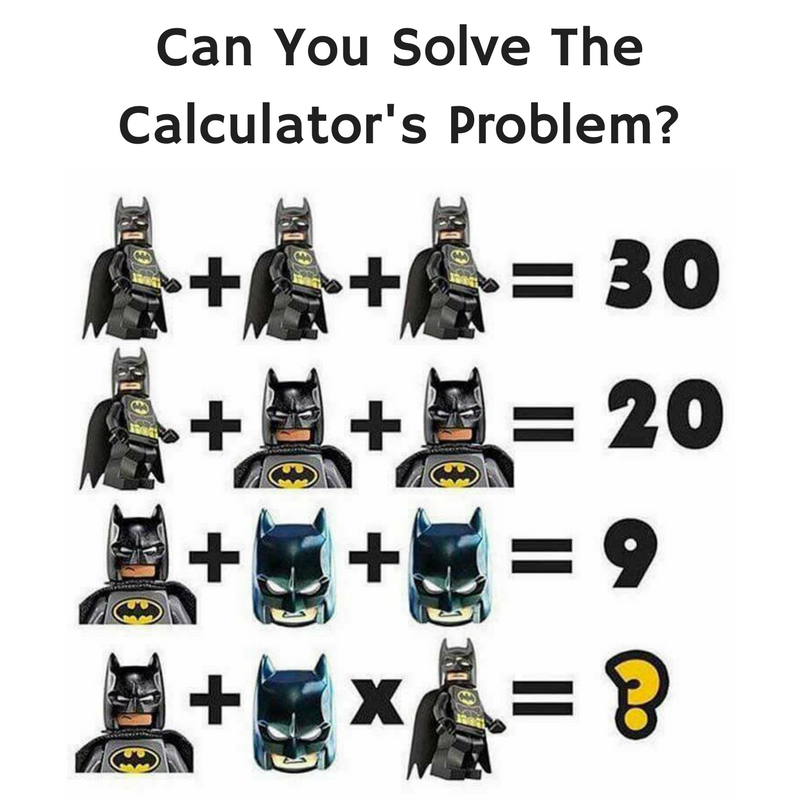Calculator has escaped from Blackgate Prison and has captured a number of Gotham citizens. He promises he will release his hostages if you can solve his complex math problem using Batman symbols!

Will you be able to solve Calculator’s complex and devious math problem?Do you think you were able to solve it?

It’s all about the order of operations.

But first we have to figure out what each of the symbols mean. The first standing Batman Lego figure is equal to 10. It’s simple algebra. 3*X=30. 30/3=X. X=10.

We can use algebra for the following 2 equations as well.

10 + 2(X) = 20. 20-10=2(X). 10=2(X). 10/2=X. X=5.

Let’s move onto the third equation.

5+2(X)=9. 2(X)=9-5. 2(X)=4. X=4/2. X=2.

Now that we have figured out what each symbol means, we can plug them into the final equation.

5+2*10=X. This is where order of operations comes into play. The order of operations is commonly known as PEMDAS. It stands for Parentheses, Exponents, Multiplication and Division, and Addition and Subtraction. It also explains which calculations you are supposed to do first.

We know that multiplication must come first. Thus the next step in our equation would be 5+20=X because we would multiple 2 times 10 to give us 20. Then we have a simple addition problem. 5 plus 20 gives us our final answer of 25.

Were you able to solve Calculator’s devious math problem?

Share with your friends and family to see if they can solve Calculator’s problem and rescue the citizens of Gotham from his vile clutches!